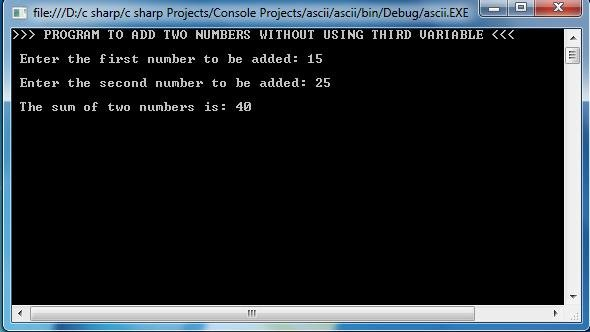# C# PROGRAM TO ADD TWO INTEGERS WITHOUT USING THIRD VARIABLE

### C# Program To Add Two Numbers/Integers without using Third Variable :

```using System;

namespace CsharpPrograms
{
class Program
{
static void Main(string[] args)
{

int x,y;
Console.WriteLine(">>> PROGRAM TO ADD TWO NUMBERS WITHOUT USING THIRD VARIABLE <<< ");
Console.Write("\n Enter the first number to be added: ");
x=Convert.ToInt32(Console.ReadLine());         // taking x value from keyboard
Console.Write("\n Enter the second number to be added: ");
y = Convert.ToInt32(Console.ReadLine());         // taking y value from keyboard
x = x + y;     /*assining the value of sum of x and y to x*/

Console.WriteLine("\n The sum of two numbers is: "+x);    /*printing the sum.*/
}
}
}```

Sample Output:For C Program : c program to add two numbers without using third variable

## 1 thought on “C# PROGRAM TO ADD TWO INTEGERS WITHOUT USING THIRD VARIABLE”

1.Sudhir DBAKings says: